What is the root mean square speed of an As4 particle as it is sublimed? (Assume at high temperatures arsenic acts like an ideal gas; the Bo

Question

What is the root mean square speed of an As4 particle as it is sublimed? (Assume at high temperatures arsenic acts like an ideal gas; the Boltzmann constant, kB, can be approximated as 1.4 x 10-23 J∙K-1) and the kinetic energy of the gas is equal to 3/2kB T

in progress 0
5 months 2021-08-04T12:01:44+00:00 1 Answers 26 views 0

1. Answer: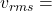273m/s

Explanation: Root Mean Square Speed of an atom or molecule is the speed of a particle in a gas. It is the average speed a particle in a gas can have.

It can be calculated: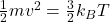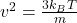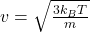m is mass of one atom or molecule in kg.

An atom of Arsenic sublimes at 614°C. Converting to Kelvin:

T = 614 + 273 = 887K

Molecular mass of As4 is approximately 0.3kg.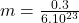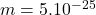kg

Calculating Root mean square speed :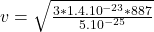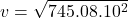v = 273m/s

The root mean square speed of As4 is approximately 273m/s.